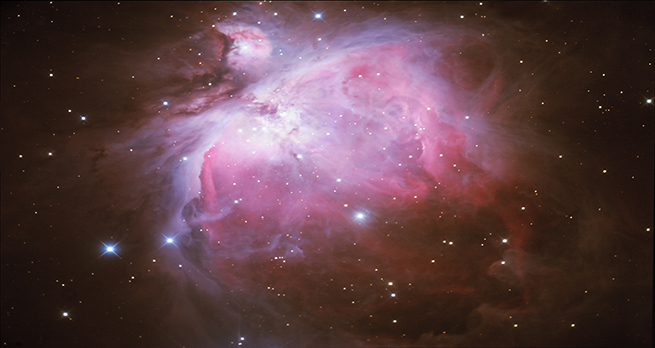In the night sky: Orion

Start this free course now. Just create an account and sign in. Enrol and complete the course for a free statement of participation or digital badge if available.

Free course

# 2.1.4 Size of a nebula

How big is the Orion Nebula? Or at least, how far across is it?Skip transcript

#### Transcript

NARRATOR:
Converting angular size to actual size Important note: for this calculation to work, the angle must be converted from degrees, minutes and seconds to radians, where 1 radian equals 57 degrees.
So 1 degree equals 1 over 57 radians
Angular size equals actual size over distance
Actual size equals distance multiplied by angular size
The Orion Nebula is 1 degree across equalling 1 over 57 radians
The Orion Nebula is 15.2 thousand million million kilometres away
The actual size of the Orion Nebula is 15.2 thousand million million multiplied by 1 over 57 kilometres
The Orion Nebula is 267 million million kilometres or 167 million million miles across.
So, very big!
End transcript

Interactive feature not available in single page view (see it in standard view).

Astronomers have a different way of estimating the size of objects, using the angle that the top and bottom of an object appears to make with the eye. This is shown in Figure 5 where the plant, the bush and the tree, while all at different distances from the observer, make the same angle at the eye.Figure 5

The observed angular size of an object depends on the size of the object and its distance from the observer.

The further away something is, the smaller it appears – and the smaller the angle it will make with the eye. Although the objects studied by astronomers are very large, they are at such vast distances away that their angular sizes are often very small. These small angles could be written as fractions of a degree, but, in practice, subdivisions of degrees are used, known as ‘minutes of arc’ (or arcmin) and ‘seconds of arc’ (or arcsec). A degree can be divided into 60 minutes of arc, and a minute of arc can be further divided into 60 seconds of arc. A single tick mark is used to represent arcmin, so 1/60 of a degree is written 1’. A double tick mark denotes arcsec, so 1/3600 of a degree (1/60 of an arcmin) is written 1”.

The Orion Nebula is about 60 arcminutes (60’) across. What does this mean? Again, we can convert this measurement to kilometres or miles, as shown in the video above.

So the Orion Nebula is about 267 million million kilometres, or 167 million million miles across – I don’t know about you – but I have difficulty in getting my mind around these sorts of distances and sizes!

Next, you will find out about the Trapezium cluster which forms part of the Orion Nebula.